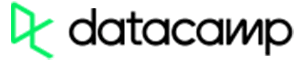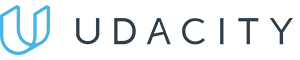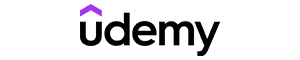Code has been added to clipboard!

# Using Python If Else Statements to Check One or More Conditions

Published Nov 25, 2019
Updated Nov 25, 2019

TL;DR – The Python if else statement executes a function based on a certain true-false condition.

## What is the Python if else statement?

The Python `if else` statement is an extended version of the `if` statement. While the Python `if` statement adds the decision-making logic to the programming language, the `if else` statement adds one more layer on top of it.

Both of them are conditional statements that check whether a certain case is true or false. If the result is true, they will run the specified command. However, they perform different actions when the set condition is false.

When an `if` statement inspects a case that meets the criteria, the statement will execute the set command. Otherwise, it will do nothing. Look at this basic example of Python if statement:

Example
``````x = 10
y = 15

if x < y:
print('X is greater than Y.')``````

Meanwhile, the `if else` statement will execute another specified action if it fails to meet the first condition.

Simply put, the `if else` statement decides this: if something is true, do something; if something is not true, then do something else.

Note: the `else` will only run when the case does not meet the condition of the `if` statement.

## If else in Python: learn the syntax

### Single condition example

For a single condition case, specify the case condition after the `if` statement followed by a colon (:). Then, fill another specified action under the `else` statement. Here's how the code structure should look like:

``if <a specific case>: <execute this command>else: <perform a different command>``

Replace `<a specific case>` with the conditions you want to examine, and `<execute this command>` with the action that should be done if the case is TRUE. Then, replace `<perform a different command>` with the actions that should be done if the case is FALSE.

Here is an example of applying Python `if else` statements. In this case, the `if` statement is printed because it fits the primary condition:

Example
``````c = 5
if(c > 4):
print("Value of c is greater than 4")
else :
print("Value of c is 4 or less")``````

In the following example, we make the code execute the `else` command by making the primary condition false:

Example
``````c = 3
if(c > 4):
print("Value of c is greater than 4")
else :
print("Value of c is 4 or less than 4")``````Pros
• Easy to use with a learn-by-doing approach
• Offers quality content
• Gamified in-browser coding experience
• The price matches the quality
• Suitable for learners ranging from beginner to advanced
Main Features
• Free certificates of completion
• Focused on data science skills
• Flexible learning timetablePros
• Simplistic design (no unnecessary information)
• High-quality courses (even the free ones)
• Variety of features
Main Features
• Nanodegree programs
• Suitable for enterprises
• Paid Certificates of completionPros
• Easy to navigate
• No technical issues
• Seems to care about its users
Main Features
• Huge variety of courses
• 30-day refund policy
• Free certificates of completion

### Checking multiple conditions with if else and elif

To check multiple `if` conditions, you can use the Python `elif` in the middle of the `if else` function instead of creating a lot of `if` statements as a big loop. The code will look like this:

Note: `elif` is short for `else if`.

``if <a specific case>: <do this statement>elif <a different case>: <execute another statement>else: <do something else>``

Replace `<a different case>` with the second condition you want to use, and `<execute another statement>` with the action that should be carried out if the second case is TRUE. Then, the rest goes the same as with the single `if else` condition example we mentioned first.

The following example shows the use of Python `if else` and also `elif`:

Example
``````x = int(input("Please input the weight of your package."))
if x >= 120:
print('This size exceeds our limitations.')
elif x >= 50:
print('You need to pay a fee of \$20 for this package.')
else:
print("There is no extra charge for the delivery.")``````

The program above will run once. If you need to run it multiple times, you would need to use `for` or while statements.

Note: Python does not use curly brackets ({}) to mark code initiation. Instead, it uses indentation.

## A common if else use in Python

Python `if else` statement is widely used for sorting comparisons of operators: greater than, less than, equal to, greater than or equal to, less than or equal to, etc.

Let's say you want to evaluate some examination results. You want to automatically convert the score numbers into letters. The code would look like this:

Example
``````x = int(input("What is your exam score?"))
if x >= 85:
print('You got an A! Congrats!')
elif x >= 75:
print('You got a B! Well done!')
elif x > 50:
print('You got a C. Not great, not terrible.')
elif x == 50:
print('You got a D. But you can do better!')
else:
print("You did not pass the exam. See the teacher for more information.")``````

• The function will output A if the score is greater than or equal to 85.
• If the score is greater than or equal to 75, it will output B.
• It will display C if the score is greater than 50.
• D will be shown if the score is equal to 50.
• If the score is less than 50, the function will print that the student did not pass his/her exam.

## Python if else: useful tips

• Always use a colon (:) at the end of every `if` line.
• You can add as many Python `elif` conditions as you want to check more options.

Days
Hours
Minutes
Seconds